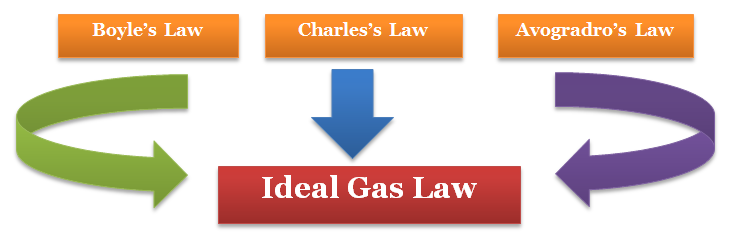Click to Chat

1800-1023-196

+91-120-4616500

CART 0

• 0

MY CART (5)

Use Coupon: CART20 and get 20% off on all online Study Material

ITEM
DETAILS
MRP
DISCOUNT
FINAL PRICE
Total Price: Rs.

There are no items in this cart.
Continue ShoppingIdeal Gas Law & Dalton’s Law of Partial Pressure

Table of Content

Combined Gas Equation

Ideal Gas Equation

Dalton’s Law of Partial Pressure

Partial Pressure in terms of Mole Fraction

Related Resources

Combined Gas EquationThe Boyle’s and Charles’ law can be combined to give a relationship between the three variables P, V and T.

Let a certain amount of a gas in a vessel have a volume V1, pressure P1 and temperature T1.

On changing the temperature and pressure to T2 and P2 respectively, the gas occupies a volume V2.

Then we can write

The above relation is called the combined gas law.

In 1812, Amadeo Avogadro stated that samples of different gases which contain the same number of molecules (any complexity, size, shape) occupy the same volume at the same temperature and pressure. It follows from Avogadro’s hypothesis that V µ n (when T and P are constant).

Ideal Gas EquationRefer to the following video for Ideal Gas Equation

A gas that would obey Boyle’s , Charle’s law  and Avogadro’s law under the conditions of temperature and pressure is called an ideal gas.

Here, we combine four measurable variables P, V, T and n to give a single equation.

V ∝ n [P, T constant]

V ∝ T [n, P constant]

Charle’s law

V ∝ 1/P [n, T constant]

Boyle’s law

The combined gas law can be written as

V ∝ nT / p

or pV ∝ nT

pV = nRT

This is called ideal gas equation

where R is the constant of proportionality or universal gas constant

The value of R was found out to be

R = 8.314 J mol–1 K–1

R = 0.0821 litre atm K–1 mol–1

R = 2 cal K–1 mol–1

Relation between molar mass and density (From ideal gas equation)

Ideal gas equation is a relation between four variables and it describes the state of any gas, therefore, it is also called equation of state.

Ideal gas is a hypothetical concept. Real gases behave ideally only under certain conditions (i.e. high temperature and low pressure).

Example 1:

Question:

What is the increase in volume when the temperature of 600 ml of air increases from 27°C to 47°C under constant pressure?

Solution:

Charle’s law is applicable as the pressure and amount remains constant.

V1 / T1 = V2 / T1 or V­1 = T1 / T2 × V2

V1 = 320 / 300 = 640 ml

Increase in volume of air = 640 – 600 = 40

Dalton’s Law of Partial Pressure

The relation between the pressures of the mixture of non-reacting gases enclosed in a vessel to their individual pressure is described in the law.

The law was given by John Dalton in 1807.

It states that.
At constant temperature, the pressure exerted by a mixture of two or more non-reacting gases enclosed in a definite volume, is equal to the sum of the individual pressures which each gas would exert if present alone in the same volume.

The individual pressures of gases are known as partial pressures.

Mathematically
If P is the total pressure of the mixture of non-reacting gases at temperature T and volume V, and P1, P2, P3 …. represent the partial pressures of the gases, then
P = P1 + P2 + P3+ ……… (T, V are constant)

Partial Pressure in terms of Mole Fraction

Mole fraction (X) defines the amount of a substance in a mixture as a fraction of total amount of all substances.

If n =1 moles of any substance is present in n moles of the mixture, then mole fraction of the substance, X1 = n1 / n

If PN2 is the partial pressure of nitrogen the mixture of SO2 and N­2. Then

PN2 = nN2 × RT/V  (= no. of moles of N2)

and Pmixture = (nN2 + nSO2) RT / V

dividing we get,

PN2 / Pmixture = (nN2 / nN2 + NSO2) = XN2

or, PN2 = XN2 × Pmixture

Gases are generally collected over water and therefore are moist.

Pressure of dry gas can be calculated by subtracting vapour pressure of water from the total pressure of the moist gas which contains water vapours also.

Pressure exerted by saturated water vapour is called aqueous tension.

Example 2 :

Question:

A 2.5 litre flask contains 0.25 mole each of sulphur dioxide and nitrogen gas at 27°C. Calculate the partial pressure exerted by each gas and also the total pressure.

Solution:

Partial pressure of SO2

PSO2 = nRT / V = 0.25 × 8.314 × 300 / 2.5 × 10–3   = 2.49 × 105 Nm–2 = 2.49 × 105 Pa

Similarly PN2 = 2.49 × 105 Pa

Following Dalton’s Law

PTotal = PN2 + PSO2   = 2.49 × 105 Pa + 2.49 × 105 Pa   = 4.98 × 105 PaQuestion 1: Which of the following expressions for Ideal gas equation is incorrect?

a. pV = nRT

b. p = nRT/V

c. P = RT/M

d. V1 / T1 = V2 / T1

Question 2: Which of the following units of R is incorrect?

a. J mol–1 K–1

b. litre atm K–1 mol–1

c. cal K–1 mol–1

d. Kg K–1 mol–1

Question 3: What would be the total vapour pressure of the mixtures of two gas A and B if mole fraction of and partial vapour pressure of gas A is 0.5 bar and 0.1 respectively.

a. 0.5 bar

b. 0.1 bar

c. 5.0 bar

d. 1.0 bar

Question 4: How many number of moles of gas A is present in a vessel of 5 L at 1 atm temperature and 273 K temperature. (R = 0.0821 litre atm K–1 mol–1  )

a. 12.2

b. 1.12

c. 11.2

d. 1.21Q.1

Q.2

Q.3

Q.4

d

d

c

a

Related Resources

Look here for Reference books of  Chemistry

You can also refer States of matter

To read more, Buy study materials of States of Matter comprising study notes, revision notes, video lectures, previous year solved questions etc. Also browse for more study materials on Chemistry here.### Course Features

• 731 Video Lectures
• Revision Notes
• Previous Year Papers
• Mind Map
• Study Planner
• NCERT Solutions
• Discussion Forum
• Test paper with Video Solution